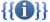# قالب:Chembox StructureTemplate documentation[view]  [history] [purge]

This box can be used as a module in the {{chembox new}}. Copy the left column into an existing chembox new, before the first section, and give the section a unigue number (the first line becomes e.g. "| Section8 = {{Chembox Structure", there are 15 sections available).

 ```| Section = {{Chembox Structure | CrystalStruct = | SpaceGroup = | Coordination = | LattConst_a = | LattConst_b = | LattConst_c = | LattConst_alpha = | LattConst_beta = | LattConst_gamma = | MolShape = | OrbitalHybridisation = | Dipole = }}``` ```| Section = {{Chembox Structure | CrystalStruct = | SpaceGroup = | Coordination = | LattConst_a = | LattConst_b = | LattConst_c = | LattConst_alpha = | LattConst_beta = | LattConst_gamma = | MolShape = | OrbitalHybridisation = | Dipole = }}```